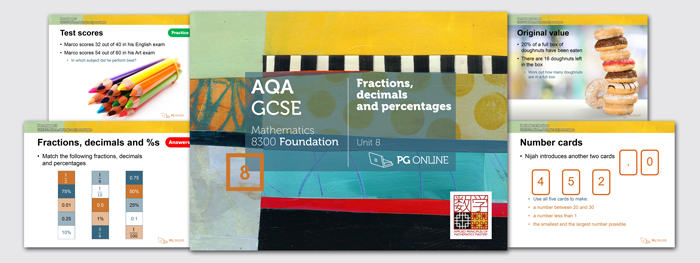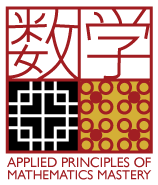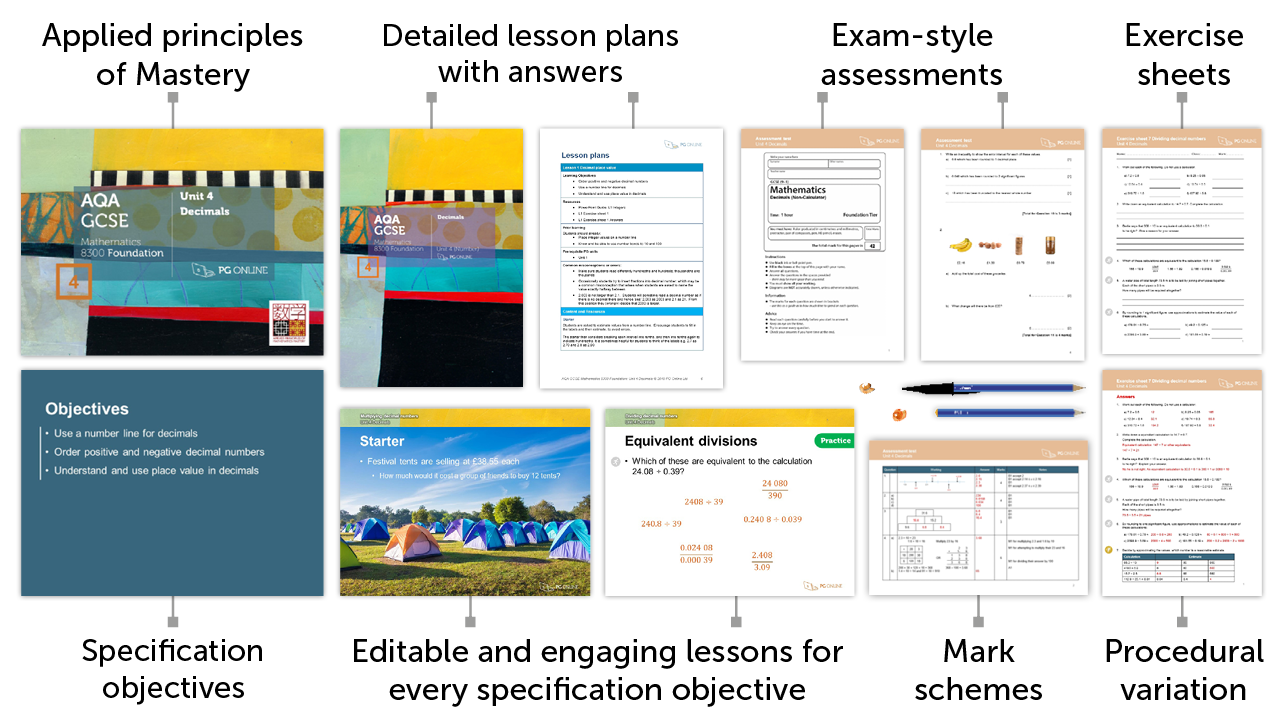# Mastery for GCSE AQA

### £75.00 + VAT+

8

## Inside the Unit...### Areas Covered

• Lesson 1: Decimals to fractions
• Lesson 2: Fractions to decimals
• Lesson 3: Percentages 1
• Lesson 4: Percentages 2
• Lesson 5: Problems involving percentages
• Lesson 6: Percentage increase
• Lesson 7: Percentage decrease
• Unit assessment

There are 7 exercise sheets and an examination-style assessment test, each with answers included in this unit.

## Mathematics Mastery

Our resources apply the principles of Mastery throughout, creating a rich and deep learning experience. Find out more about Mastery with our quick guide.## Summary

This unit focuses on the properties and applications of fractions, decimals and percentages. Decimal and fractions are considered first with an emphasis on developing a mastery of these dual concepts. From here, percentages are introduced; expressing an amount as a percentage, converting between fractions, decimals and percentages and comparing two quantities using percentages. In context, percentages are interpreted as operators, percentages of amounts are found and reasoning tasks involving percentages are posed. Fluency with percentages grows as the unit moves on to consider percentage increase and decrease; using a decimal multiplier to represent the percentage change and to work in reverse. Problems in context involving percentage change are posed and solved.

## What's included in the toolkit?The GCSE units have been written to satisfy the specification for the AQA 8300 Maths GCSE. Each unit contains:

• PowerPoint slides for each component
• Detailed lesson plans
• Learning objectives and outcomes
• Worksheets and homework activities with answers
• End-of-unit, exam-style assessment test with answers
• Other material and links to online resources

## What people say...

Professional looking resources that would enable consistency within the department and the use of worksheet and homework task that comprehensively covered the spec points.

Helen Webb. Science teacher, Lutterworth College

The resources were very good.

James Knightbridge. Mathematics teacher, Clayesmore School

## Inside the Unit...

### Areas Covered

• Lesson 1: Decimals to fractions
• Lesson 2: Fractions to decimals
• Lesson 3: Percentages 1
• Lesson 4: Percentages 2
• Lesson 5: Problems involving percentages
• Lesson 6: Percentage increase
• Lesson 7: Percentage decrease
• Unit assessment

There are 7 exercise sheets and an examination-style assessment test, each with answers included in this unit.

## Mathematics Mastery

Our resources apply the principles of Mastery throughout, creating a rich and deep learning experience. Find out more about Mastery with our quick guide.• ### How to order

1. Add individual units to a draft order or download a blank order form below to complete manually

2. Using a draft order you can either:

2. Email us your complete order
3. Create a PDF
(to fax or email at a later date)

• ### Unit Pricing and Discounts

Each unit is individually priced for LIFE. Please view each unit for detail.

Discounts are cumulative based on the total of units licenced to your department.

All prices subject to further discounts and VAT.

There are no annual renewal fees.

 2-5 units 10% discount 6-11 units 15% discount 12-23 units 20% discount 24+ units 25% discount